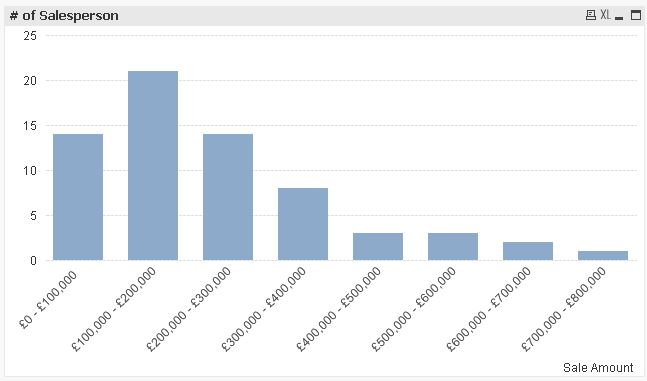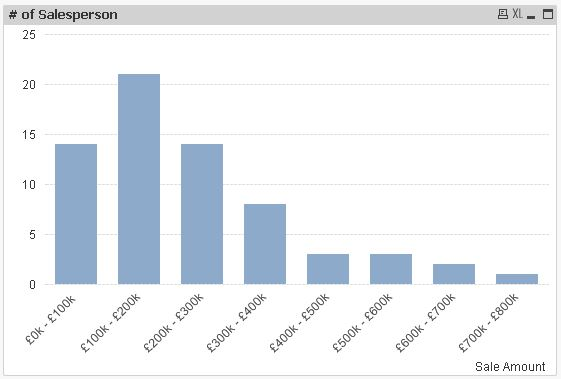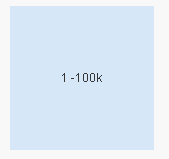# New to QlikView

Discussion board where members can get started with QlikView.

Announcements
BARC’s The BI Survey 19 makes it official. BI users love Qlik. GET REPORT
HighlightedNot applicable

## How do I group values in a bar chart ?

Hi,

What is the best method for summing data records into bands ? In the script ? Or in set analysis ?

I have thousands of transactions of sales data made up of the following fields....

Sale ID

Date of Sale

Sale Amount (in £)

Salesperson

I want to create a simple bar chart showing the number of salespersons ('Y' axis) who have a total sales figure between £0 and £100,000; between £100,001 and £200,000; between £200,001 and £300,000....and so on, in groups or bands of £100,000. The bands will appear on the 'X' axis.

So, for example, Salesman A who has sales of £650,000 will count as 1 salesperson in the £600,001 - £700,000 column band of the chart.

How should I approach this?

MV

Tags (1)
1 Solution

Accepted SolutionsMVP

## Re: How do I group values in a bar chart ?

Hi,

maybe like this:```=Aggr(Dual(Money(SubField(Class(Sum([Sale Amount]),100000),' <= x < ',1),'£#,##0')&' - '&Money(SubField(Class(Sum([Sale Amount]),100000),' <= x < ',2),'£#,##0'),Class(Sum([Sale Amount]),100000)),Salesperson)
```

or this:```=Aggr(Dual(Money(SubField(Class(Sum([Sale Amount]),100000),' <= x < ',1)/1000,'£#,##0k')&' - '&Money(SubField(Class(Sum([Sale Amount]),100000),' <= x < ',2)/1000,'£#,##0k'),Class(Sum([Sale Amount]),100000)),Salesperson)
```

hope this helps

regards

Marco

11 RepliesMVP

## Re: How do I group values in a bar chart ?

look at class function

from Qlik help

class(expression, interval [ , label [ , offset ]])

Creates a classification of expressions. The bin width is determined by the number set as interval. The result is shown as a<=x<b, where a and b are the upper and lower limits of the bin. The x can be replaced by an arbitrary string stated in label. 0 is normally the default starting point of the classification. This can be changed by adding an offset.

Examples:

class( var,10 ) with var = 23 returns '20<=x<30'

class( var,5,'value' ) with var = 23 returns '20<= value <25'

class( var,10,'x',5 ) with var = 23 returns '15<=x<25'

in bar chart you can use this as calculated dimension

class([Sale Amount], 100000)

and this as expression

count(Salesperson)Partner

## Re: How do I group values in a bar chart ?

hi

you do it like this

in the dimension you use calculate dimension

with this expression :

class(aggr(sum([Sale Amount (in £)]),Salesperson),'X',100000)

and to count the sales persons use this expression

count(distinct Salesperson)Not applicable

## Re: How do I group values in a bar chart ?

Thanks Massimo,

Is there any way to display the x-axis labels differently. The first label on my chart is being displayed like this:

1 <=x<100001

Can I edit it like this....

1 - 100k

or this....

£1 - £100kMVP

## Re: How do I group values in a bar chart ?

You might have to play around with it a little, try something like this as your calculated dimension:

=SubField(Class(), '<', 1) & '-' & Floor(SubField(Class(), '<', 3)/1000) & 'k' // Use the class function you have right now within the SubField function

For example, this in a text box

=SubField('1 <=x<100001', '<', 1) & '-' & Floor(SubField('1 <=x<100001', '<', 3)/1000) & 'k'

gives this:HTH

Best,

SunnyMVP

## Re: How do I group values in a bar chart ?

Or

Aggr(Class(Sum(...

Regards

Marco

Honored Contributor

## Re: How do I group values in a bar chart ?

Dear Maureen,

Use replace() function for replacing '<= x <' with '-'.

=Replace(Class([Sale Amount], 100000),'<= x <', '-')

Regards,

NeethaMVP

## Re: How do I group values in a bar chart ?

Hi,

maybe like this:```=Aggr(Dual(Money(SubField(Class(Sum([Sale Amount]),100000),' <= x < ',1),'£#,##0')&' - '&Money(SubField(Class(Sum([Sale Amount]),100000),' <= x < ',2),'£#,##0'),Class(Sum([Sale Amount]),100000)),Salesperson)
```

or this:```=Aggr(Dual(Money(SubField(Class(Sum([Sale Amount]),100000),' <= x < ',1)/1000,'£#,##0k')&' - '&Money(SubField(Class(Sum([Sale Amount]),100000),' <= x < ',2)/1000,'£#,##0k'),Class(Sum([Sale Amount]),100000)),Salesperson)
```

hope this helps

regards

MarcoNot applicable

## Re: How do I group values in a bar chart ?

Thanks to everyone who helped. I tried out each of your solutions on my application.

I do like Marco's though ! Very well explained with an example application and additional screenshots.

That's a great help Marco

MVNot applicable

## Re: How do I group values in a bar chart ?

One more thing Marco. How do I show those groups/bands on the x axis which have a value of 0 ?